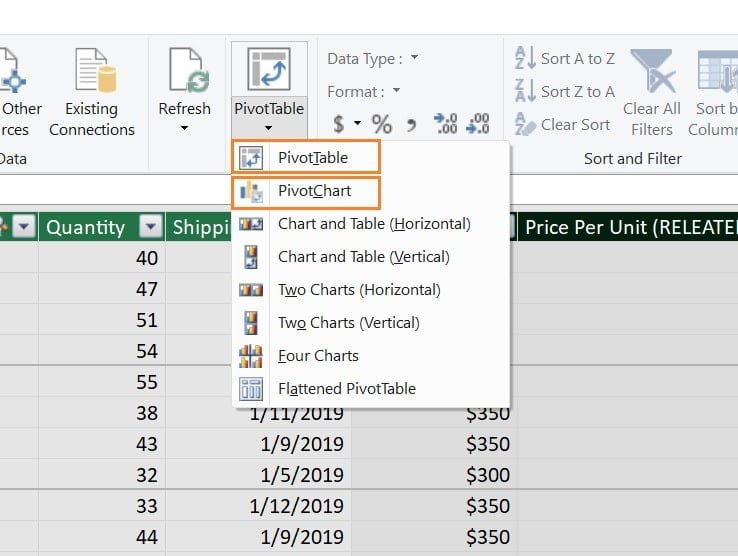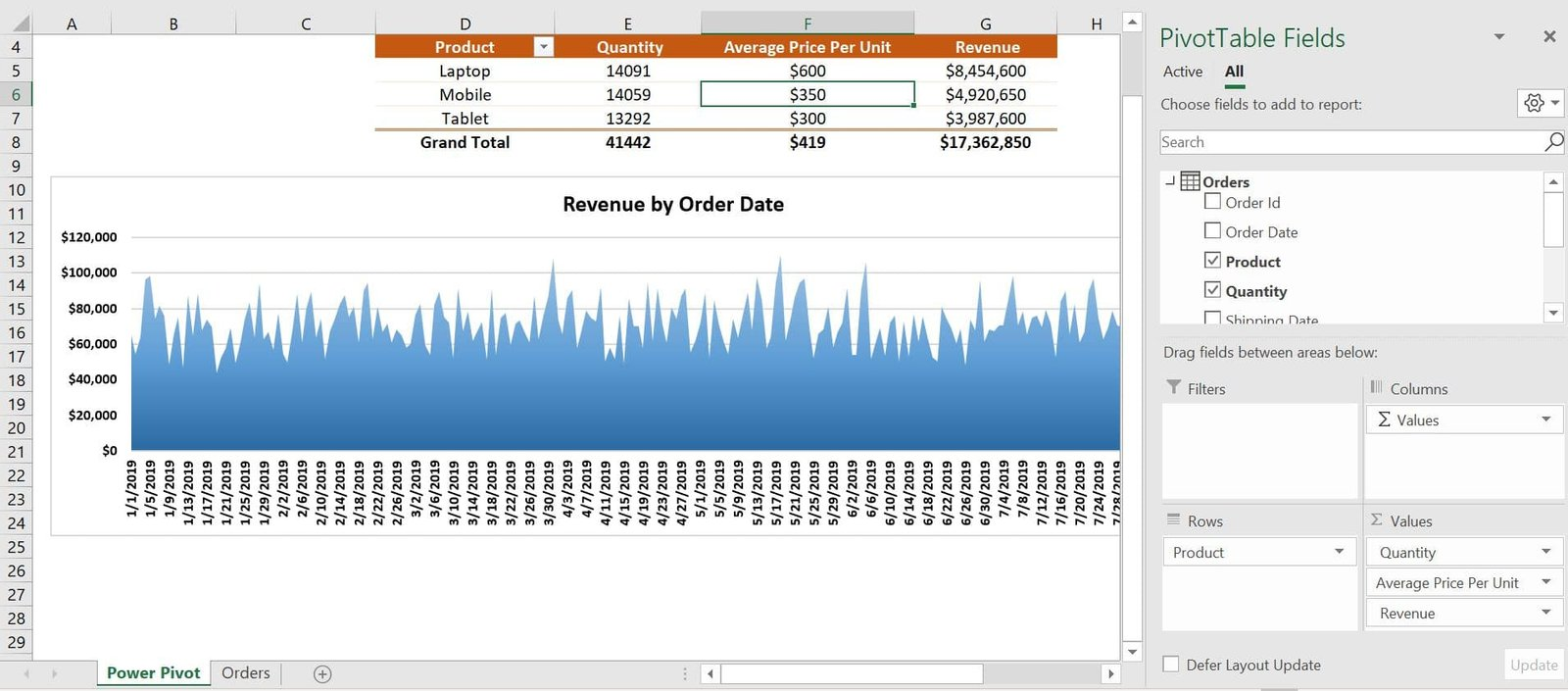Home>Blogs>Power Pivot>Calculated Column in Power Pivot (LOOKUPVALUE Vs RELATED)

# Calculated Column in Power Pivot (LOOKUPVALUE Vs RELATED)

In this article, we have explained how to use calculated column in Power Pivot Data Model.  We have taken the example of Orders data wherein we have product level Unit Sold and we have to add the Calculated column for Revenue. To calculate the revenue we need the Price Per Unit on product level which we have in Product Master table. So first we have get the Price Per Unit in Orders table.

We have used two different method to get the Price Per Unit in Orders table from Product Master table.

## LOOKUPVALUE function:

LOOKUPVALUE function returns the value in result_columnName for the row that meets all criteria specified by search_columnName and search_value.

### Syntax:

`LOOKUPVALUE( <result_columnName>, <search_columnName>, <search_value>[, <search_columnName>, <search_value>]…[, <alternateResult>])`
• result_columnName: The name of an existing column that contains the value you want to return. The column must be named using standard DAX syntax, usually, fully qualified. It cannot be an expression.
• search_columnName: The name of an existing column, in the same table as result_columnName or in a related table, over which the look-up is performed. The column must be named using standard DAX syntax, usually, fully qualified. It cannot be an expression.
• search_value: A scalar expression that does not refer to any column in the same table being searched.
• alternateResult: (Optional) The value returned when the context for result_columnName has been filtered down to zero or more than one distinct value. When not provided, the function returns BLANK() when result_columnName is filtered down to zero value or an error when more than one distinct value.

Below is the LOOKUPVALUE formula which we have used in this example:

`=LOOKUPVALUE('Product Master'[Price per unit],[Product],[Product])`

## RELATED function:

Second method we have used to get the Price Per Unit from Product Master to Orders table is “RELATED” function. The RELATED function requires that a relationship exists between the current table and the table with related information. You specify the column that contains the data that you want, and the function follows an existing many-to-one relationship to fetch the value from the specified column in the related table. If a relationship does not exist, you must create a relationship.

So we have created the relationship between Orders table and Product Master table.

### Syntax of RELATED Function:

`RELATED(<column>)`

Column: The column that contains the values you want to retrieve.

In this example we have used below formula:

`=RELATED('Product Master'[Price per unit])`

Once we have got the Price Per Unit in Orders then we can easily calculate the Revenue. We have used below given formula in Power Pivot Data Model to calculate the Revenue:

`=[Quantity]*[Price Per Unit]`

Now our data model part is completed, we can click on the Pivot Table button to create the pivot table. We have created below given Power Pivot and Pivot Chart:Below is the Pivot table and Pivot chart which we have created: